# CBSE Term 1 Class 10 Science Set-4, Board Exam 2021-22: Question Paper and Answer Key

CBSE 2021-22, Class 10 Science board today's exam question paper. Check the answers key of the class 10 Science (Term 1) paper.

## Class 10th CBSE Term 1, Science SET – 4 Board Exam Question Paper and Answers Key 2021-22 Table

• SUBJECT- Science (Theory)
• Term- I
• Time allowed- 90 minutes
• Maximum Marks- 40

## 1. A student took Sodium Sulphate solution in a test tube and added Barium Chloride solution to it. He observed that an insoluble substance has formed. The colour and molecular formula of the insoluble substance is

• Grey, Ba2SO4
• Yellow, Ba(SO4)2,
• White, BaSO4
• Pink, BaSO4,

## 2. Which of the following oxide(s) is/are soluble in water to form alkalis?

(i) Na2O (ii) SO2 (iii) K2O (iv) NO2

• (i) and (iii)
• (i) only
• (ii) and (iv)
• (iii) only

## 3. Study the diagram given below and identify the gas formed in the reaction.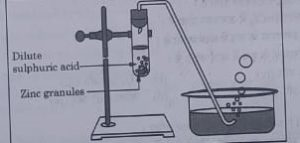• Carbon di-oxide which extinguishes the burning candle.
• Oxygen due to which the candle burns more brightly.
• Sulphur dioxide which produces a suffocating smell.
• Hydrogen which while burning produces a popping sound.

## 4. Sodium reacts with water to form sodium hydroxide and hydrogen gas. The balanced equation which represents the above reaction is

• Na(s) + 2H20(l) → 2NaOH(aq) + 2H2(g)
• 2Na(s) + 2H2O(l) → 2NaOH(aq) +H2(g)
• 2Na(s) + 2H20(l) → NaOH(aq) + 2H2(g)
• 2Na(s) + H20(l) – 2NaOH(aq) + 2H2(g)

## 5. Which of the options in the given table are correct? Option | Natural Source | Acid Present

1. Orange | Oxalic acid
2. Sour milk | Lactic acid
3. Ant sting | Methanoic acid
4. Tamarind | Acetic acid
• (i) and (ii)
• (i) and (iv)
• (ii) and (iii)
• (ii) and (iv)

## 6. C6H12O6 (aq) + 602(aq) →6CO2(aq) + 6H2O The above reaction is a/an

• displacement reaction
• endothermic reaction
• exothermic reaction
• neutralisation reaction

## 7. Which of the following statements about the reaction given below are correct? MnO2 + 4HCI → MnCl2 +2H20 + Cl2

1. HCl is oxidized to Cl2
2. MnO2 is reduced to MnCl2
3. MnCl2 acts as an oxidizing agent
4. HCl acts as an oxidizing agent
• (i), (iii) and (iv)
• (i), (ii) and (iii)
• (i) and (ii) only
• (iii) and (iv) only

## 8. Select from the following the statement which is true for bases.

• Bases are bitter and turn blue litmus red.
• Bases have a pH less than 7
• Bases are sour and change from red litmus to blue.
• Bases turn pink when a drop of phenolphthalein is added to them.

## 9. Study the following table and choose the correct option: Salt – Parent Acid – Parent Base – Nature of Salt

• Solidum Chloride – HCI – NaOH – Basic
• Sodium Carbonate – H2CO3 – NaOH – Neutral
• Sodium Sulphate – H2SO4 – NaOH – Acidic
• Sodium Acetate – CH3COOH – NaOH – Basic

## 10. It is important to balance the chemical equations to satisfy the law of conservation of mass. Which of the following statements of the law is incorrect?

• The total mass of the elements present in the reactants is equal to the total mass of the elements present in the products.
• The number of atoms of each element remains the same, before and
after a chemical reaction.
• The chemical composition of the reactants is the same before and after the reaction
• Mass can neither be created nor can it be destroyed in a chemical
Reaction.

## 11. Consider the following statements in connection with the functions of the blood vessels marked A and B in the diagram of a human heart as shown.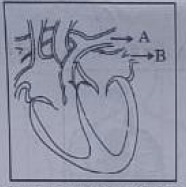1. Blood vessel A – It carries carbon dioxide rich blood to the lungs.
2. Blood vessel B – It carries oxygen rich blood from the lunga.
3. Blood vessel B – Left atrium relaxes as it receives blood from this
blood vessel
4. Blood vessel A – Right atrium has thick muscular wall as it has to
pump blood to this blood vessel.

The correct statements are

• (a) (i) and (ii) only
• (i) and (iii) only
• (i), (iii) and (iv)
• (i), (ii) and (iii)

## 12. In Living organisms During respiration which of the following Products are not formed if oxygen is not available?

• Carbon dioxide + Water
• Carbon dioxide + Alcohol
• Lactic acid + Alcohol
• Carbon dioxide + Lactic Acid

## 13. The Correct Statements with reference to single called organisms are

1. Complex substances are not broken down in to simpler substances.
2. Simple diffusion is sufficient to meet the requirement of exchange of gases.
3. Specialized tissues perform different functions in the organism.
4. Entire surface of the organism is in contact with the environment for taking in food.
• (i) and (iii)
• (ii) and (iii)
• (ii) and (iv)
• (i) and (iv)

## 14. Which one among the following is not removed as a waste product from the body of a plant?

• Resins and Gums
• Urea
• Dry Leaves
• Excess Water

## 15. Which one of the following statements are correct in reference to the role of A (Shown in the given diagram) during a breathing cycle in human beings?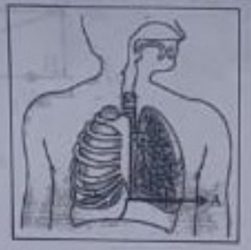1. It helps to decrease the residual volume of air in lungs.
2. it flattens as we inhale.
3. it gets raised as we inhale.
4. it helps the chest cavity to become larger.
• (ii) and (iv)
• (iii) and (iv)
• (i) and (ii)
• (i), (ii) and (iv)

## 16. Which one of the following conditions is true for the state of stomata of a green leaf shown in the given diagram?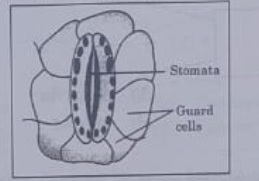• Large amount of water flows in to the ground cells.
• Gaseous exchange is occurring in large amount.
• Large amount of water flows out from the guard cells.
• Large amount of sugar collects in the guard cell

## 17. In which of the following is a concave mirror used?

• A solar cooker
• A rear view mirror in vehicles
• A safety mirror in shopping malls
• In Viewing full size image of distant tall buildings.

## 18. A student wants to obtain magnified image of an object AB as on a Screen. Which one of the following arrangements shows the correct position of AB for him/her to be successful?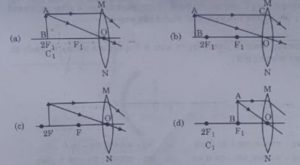## 19. The following diagram shows the use or an optical device to perform an experiment of light. As per the arrangement shown, the optical device is likely to be a;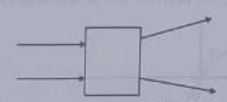• Concave mirror
• Convex mirror
• Concave lens
• Convex lens

• air-A
• A-B
• B-C
• C-air

## 21. which of the following statements is not true for scattering of light?

• Colour of the scattered tight depends on the size of particles of the atmosphere.
• Red light is least scattered in the atmosphere.
• Scattering of light Lakes place as various colours of white light travel with different speed in fir.
• The fine particles in the atmospheric air scatter the blue, light more strongly than red. So the scattered blue light enters our eyes.

## 22. For the diagram shown according to the new Cartesian sign convention the magnification of the image formed will have the following specifications: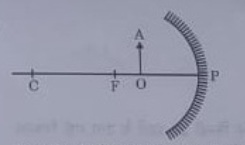• Sign – Positive, Value — Leas than 1
• Sign — Positive, value – More than 1
• Sign — Negative, Value — Less than 1
• Sign Negative, value — More than 1ospheric air scatter the blue, light more strongly than red. So the scattered blue light enters our eyes.

## 23. A ray of light is incident as shown. If A, a and C are three different transparent media. then which among the following options is true for the given Diagram?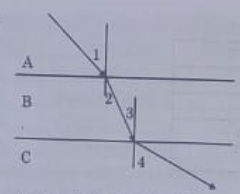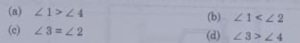## 24. In the diagram given below X and Y are the end colours of the specturm of white light. The colour of ‘Y’ Represents the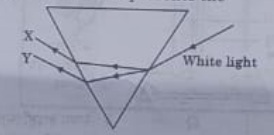• Colour of sky as seen from earth during the day.
• colour of the sky as seen from the moon.
• Colour used to paint the danger signals.
• Colour of sun at the time of noon

## 25. Which one of the following reactions is categorised as thermal decomposition reaction ?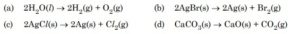## 26. Consider the pH value of the following acidic samples :

S. No. Sample pH Value
1 Lemon Juice 2.2
2 Gastric Juice 1.2
3 Vinegar 3.76
4 Dil. Acetic acid 3.0

The decreasing order of their H+ ion concentration is

• 3 > 4 > 1 > 2
• (b) 2 > 1 > 3 > 4
• 2 > 1 > 4 > 3
• 3 > 4 > 2 > 1

## 27. Study the experimental set up shown in given figure and choose the correct option from the following :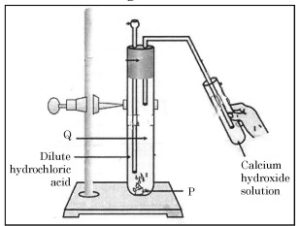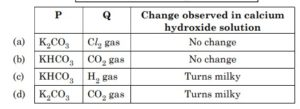## 28. Which one of the following structures correctly depicts the compound CaCl2?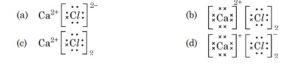## 29. The pair(s) which will show displacement reaction is/are

1. NaCl solution and copper metal
2. AgNO3 solution and copper metal
3. Al2(SO4)3 solution and magnesium metal
4. ZnSO4 solution and iron metal
• (ii) only
• (ii) and (iii)
• (iii) and (iv)
• (i) and (ii)

## 30. Which of the following salts do not have the water of crystalisation ?

1. Bleaching Powder
2. Plaster of Paris
3. Washing soda
4. Baking soda
• (ii) and (iv)
• (i) and (iii)
• (ii) and (iii)
• (i) and (iv)

### The correct answer is (i) and (iv)

Question No. 31-35 consists of two statements – Assertion (A) and Reason (R).
Answer these questions selecting the appropriate option given below :

• Both (A) and (R) are true and (R) is the correct explanation of (A).
• Both (A) and (R) are true but (R) is not the correct explanation of (A).
• (A) is true, but (R) is false.
• (A) is false, but (R) is true.

## 36. A student was asked to write a stepwise procedure to demonstrate that carbon dioxide is necessary for photosynthesis. He wrote the following steps. The wrongly worded step is –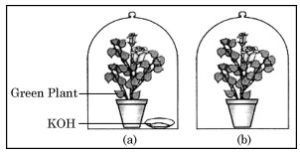• Both potted plants are kept in dark room for at least three days.
• Bottom of the bell jars is sealed to make them air tight.
• Both potted plants are kept in sunlight after the starch test.
• A leaf from both the plants is taken to test the presence of starch.

## 37. Respiratory structures of two different animals-a fish and a human being are as shown. Observe (a) and (b) and select one characteristic that holds true for both of them.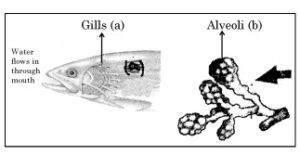• Both are placed internally in the body of animal.
• Both have thin and moist surface for gaseous exchange.
• Both are poorly supplied with blood vessels to conserve energy.
• In both the blood returns to the heart after being oxygenated

## 38. Observe the diagram of an activity given below. What does it help to conclude, when the person exhales into the test-tube ?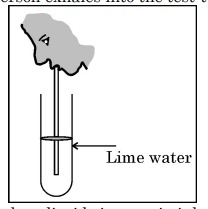• Percentage of carbon dioxide is more in inhaled air.
• Fermentation occurs in the presence of oxygen.
• Percentage of carbon dioxide is more in the exhaled air.
• Fermentation occurs in the presence of carbon dioxide.

• + 2D
• – 2D
• + 5D
• – 5D

## 40. The radius of curvature of a converging mirror is 30 cm. At what distance from the mirror should an object be placed so as to obtain a virtual image ?

• Infinity
• 30 cm
• Between 15 cm and 30 cm
• Between 0 cm and 15 cm

## 41. The length of small intestine in a deer is more as compared to the length of small intestine of a tiger. The reason for this is –

• Mode of intake of food.
• Type of food consumed.
• Presence or absence of villi in intestines.
• Presence or absence of digestive enzymes

## 42. Identify the two components of Phloem tissue that help in transportation of food in plants.

• Phloem parenchyma & sieve tubes
• Sieve tubes & companion cells
• Phloem parenchyma & companion cells
• Phloem fibres and sieve tubes

## 44. Which of the following statements is not true in reference to the diagram shown above ?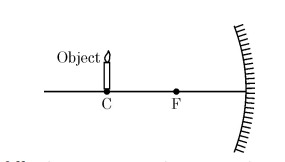• Image formed is real.
• Image formed is enlarged.
• Image is formed at a distance equal to double the focal length.
• Image formed is inverted.

## 45. In the diagram shown above n1, n2 and n3 are refractive indices of the media 1, 2 and 3 respectively. Which one of the following is true in this case ?• n1= n2
• n1>n2
• n2>n3
• n3>n1

## 46. The refractive index of medium A is 1.5 and that of medium B is 1.33. If the speed of light in air is 3 x 108 m/s, what is the speed of light in medium A and B respectively ?

• 2 x 108 m/s and 1.33 x 108 m/s
• 1.33 x 108 m/s and 2 x 108 m/s
• 2.25 x 108 m/s and 2 x 108 m/s
• 2 x 108 m/s and 2.25 x 108 m/s

• + 3.0 cm
• + 2.5 cm
• + 1.0 cm
• + 0.75 cm

## 48. 50.0 mL of tap water was taken in a beaker. Hydrochloric acid was added drop by drop to water. The temperature and pH of the solution was noted. The following graph was obtained. Choose the correct statements related to this activity.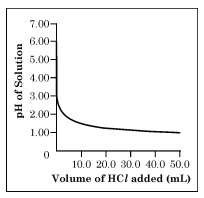1. The process of dissolving an acid in water is highly endothermic.
2. The pH of the solution increases rapidly on addition of acid.
3. The pH of the solution decreases rapidly on addition of acid.
4. The pH of tap water was around 7.0.
• and (ii)
• (i) and (iii)
• (iii) and (iv)
• (ii) and (iv)

### The correct answer is (iii) and (iv)

Section-C consists of three cases followed by questions. There are a total of 12 questions (Q. No. 49 to 60) in this section. Attempt any 10 questions from this section. The first attempted 10 questions would be evaluated.

Case-I :

A student, took four metals P, Q, R and S and carried out different experiments to study the properties of metals. Some of the observations were :

• All metals could not be cut with knife except metal R.
• Metal P combined with oxygen to form an oxide M2O3 which reacted with both acids and bases.
• Reaction with water.

P – Did not react either with cold or hot water but reacted with steam

Q – Reacted with hot water and the metal started floating

R – Reacted violently with cold water.

S – Did not react with water at all

Based on the above observations answer the following :

• P
• R
• S
• Q

• Iron
• Zinc
• Potassium
• Magnesium

• P
• Q
• R
• S

## 52. The increasing order of the reactivity of the four metals is:

• P<Q<R
• S<R<Q

• S<P<Q
• P<R<Q
•

Case-II:

The figure shown below represents a common type of dialysis called as Haemodialysis. It removes waste products from the blood. Such RB excess salts, and urea which are insufficiently removed by the kidney in patients with kidney failure. During the procedure, the patient’s blood is cleaned by filtration through a series of semi-permeable membranes before being returned to the blood of the patient. On the basis of this, answer the following questions: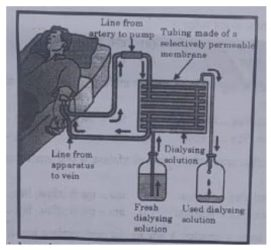## 53. The haemodialyzer has semi-permeable lining of tubes which help to:

• To maintain osmotic pressure of blood.
• To filter nitrogenous wastes from the dialyzing solution.
• In passing the waste product, in the dialyzing solution.
• To pump purified blood back into the body of the patient.

## 54. which one of the following is not a function of Artificial Kidney?

• To remove nitrogenous wastes from the blood.
• To remove excess fluids from the blood.
• To reabsorb essential nutrients from the blood.
• To filter and purify the blood.

## 55. The ‘used dialysing’ solution is rich in:

• Urea and excess salts
• Blood cells
• Lymph
• Proteins

## 56. Which part of the nephron in human kidney, serves the function of reabsorption of certain substances?

• Glomerulus
• Bowmans Capsule
• Tubules
• collecting duct

### The correct answer is Glomerulus

Case-III:

A compound microscope is an instrument which consists of two lenses L1 and L2. The lens L1 called objective, forms a real, inverted and magnified image of the given object. This serves as the object for the second lens L2; the eye piece The eye piece function like a simple microscope or magnifier. It produces the final image, which is inverted with respect to the original object, enlarged and virtual.

## 57. What types of lenses must be L1 and L2?

• Both concave
• Both convex
• L1 — concave and L2 — convex
• L1 — convex and L2 — concave

## 58. What is the value and sign of magnification (according to the new Cartesian sign convention) of the image formed by L1?

• Value = Less than 1 and Sign = Positive
• value = More than 1 and Sign = Positive
• value = Less than 1 and Sign = Negative
• value = More than 1 and Sign = Negative

## 59. What is the value and sign of (according to new Cartesian sign convention) magnification of the image formed by L2?

• value = Less than 1 and Sign = Positive
• value = More than 1 and Sign = Positive
• Value = Less than 1 and Sign = Negative
• Value = More than 1 and Sign = Negative

• 12 cm
• 16 cm
• 18 cm
• 20 cm